Ex 1.2

Chapter 1 Class 10 Real Numbers
Serial order wise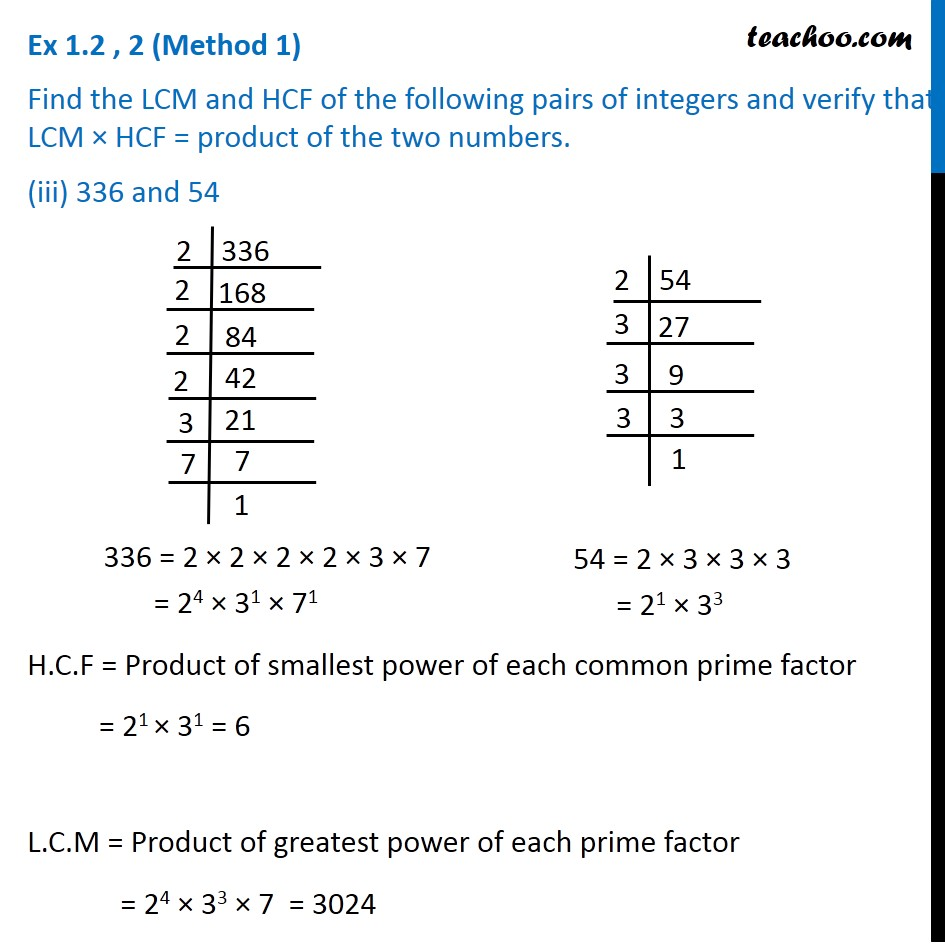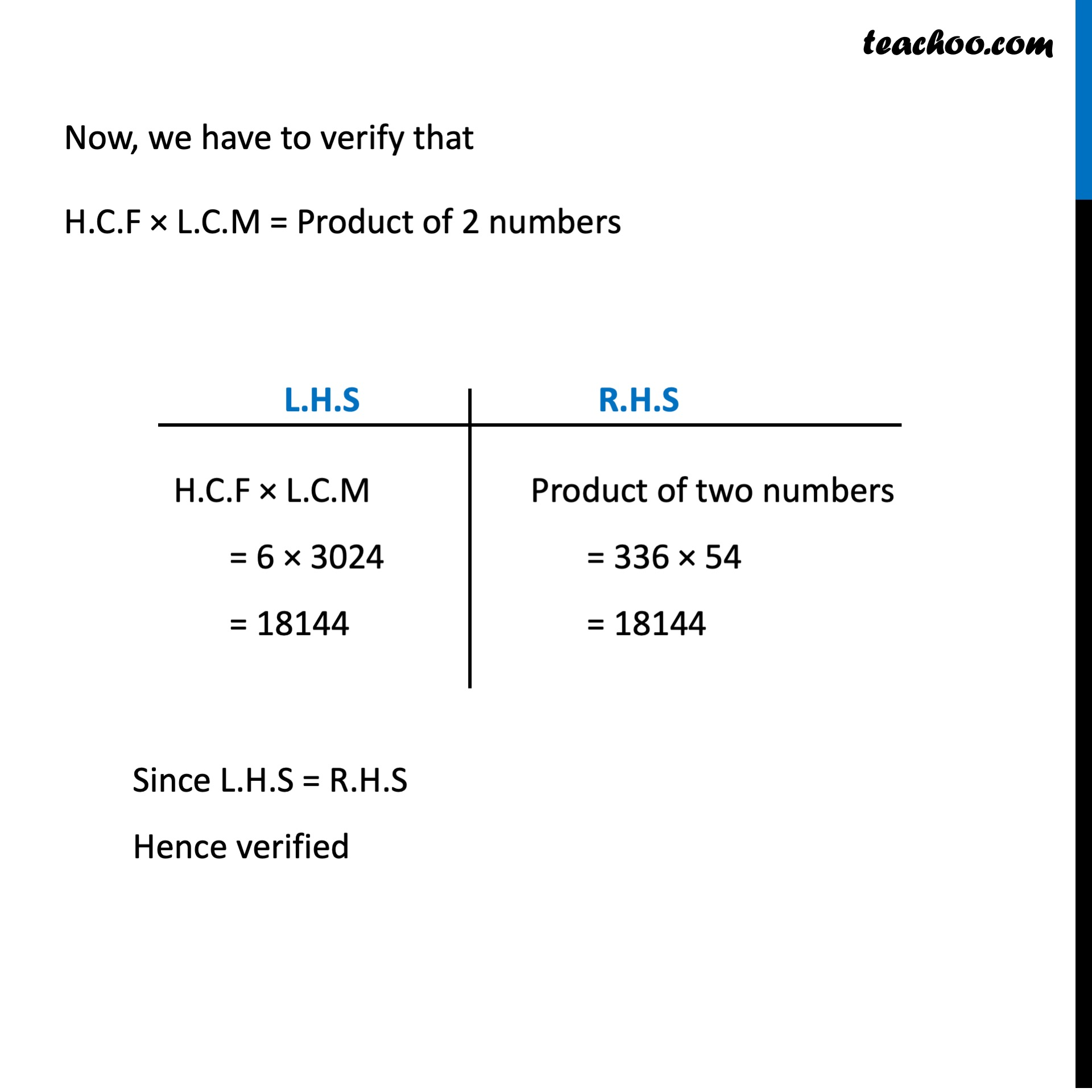This video is only available for Teachoo black users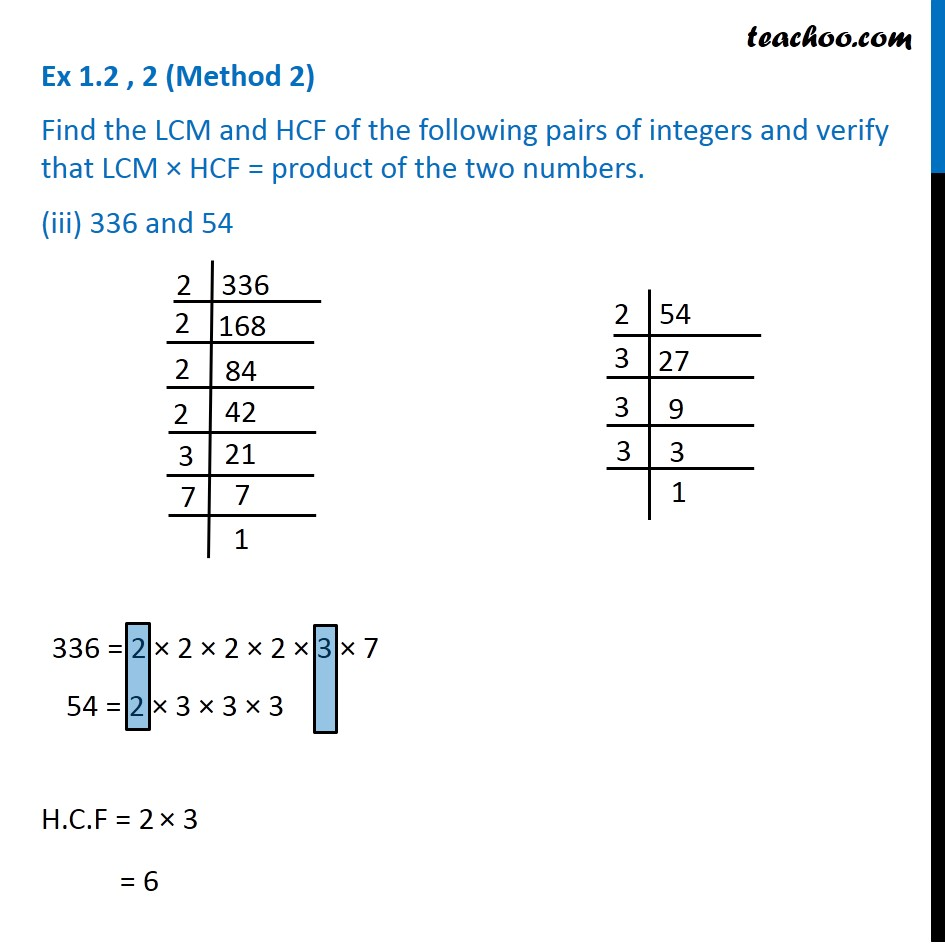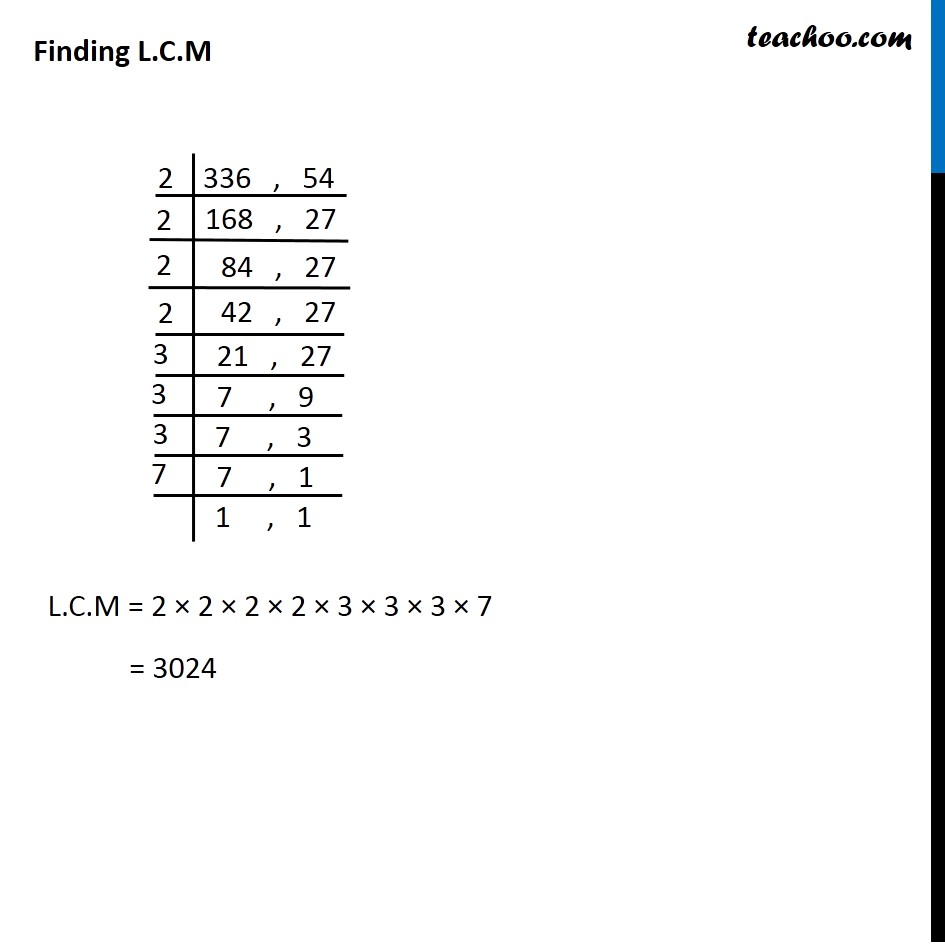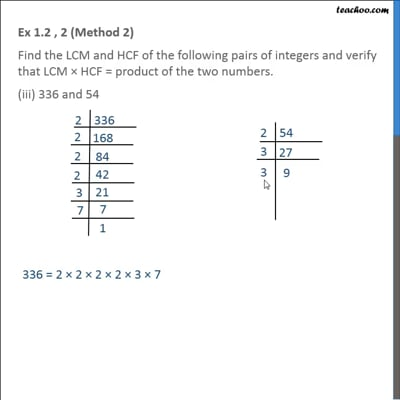This video is only available for Teachoo black users

Introducing your new favourite teacher - Teachoo Black, at only ₹83 per month

### Transcript

Ex 1.2 , 2 (Method 1) Find the LCM and HCF of the following pairs of integers and verify that LCM × HCF = product of the two numbers. (iii) 336 and 54 336 = 2 × 2 × 2 × 2 × 3 × 7 = 24 × 31 × 71 54 = 2 × 3 × 3 × 3 = 21 × 33 H.C.F = Product of smallest power of each common prime factor = 21 × 31 = 6 L.C.M = Product of greatest power of each prime factor = 24 × 33 × 7 = 3024 Now, we have to verify that H.C.F × L.C.M = Product of 2 numbers H.C.F × L.C.M = 6 × 3024 = 18144 Product of two numbers = 336 × 54 = 18144 Since L.H.S = R.H.S Hence verified Ex 1.2 , 2 (Method 2) Find the LCM and HCF of the following pairs of integers and verify that LCM × HCF = product of the two numbers. (iii) 336 and 54 336 = 2 × 2 × 2 × 2 × 3 × 7 54 = 2 × 3 × 3 × 3 H.C.F = 2 × 3 = 6 Finding L.C.M L.C.M = 2 × 2 × 2 × 2 × 3 × 3 × 3 × 7 = 3024 Now, we have to verify that H.C.F × L.C.M = Product of 2 numbers H.C.F × L.C.M = 6 × 3024 = 18116 Product of two numbers = 336 × 54 = 18116 Since L.H.S = R.H.S Hence verified